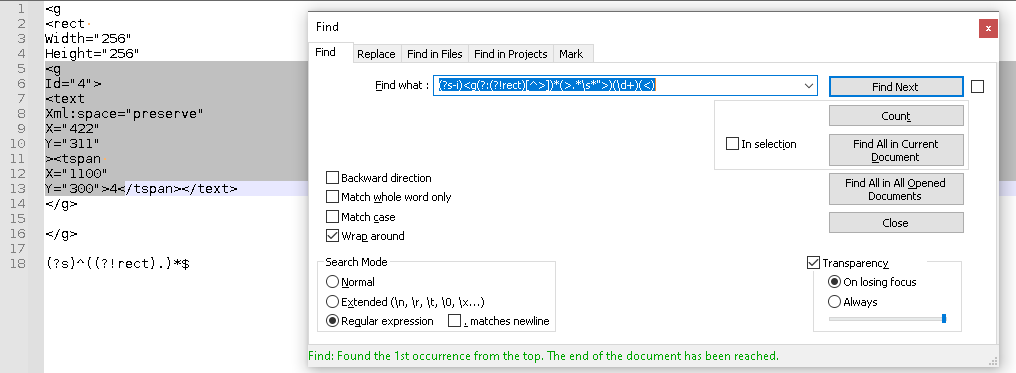# Regex help with replacement

• Hi,

I have a little bit of a problem with my regex code and was wanting to see if anyone could help. I’m trying to capture a number at the bottom of the code shown (>4< ) and replace it at the top of the code where the id=“4” with xname=“4” xid = “4”. The code is shown below.

``````<g
Id="4">
<text
Xml:space="preserve"
X="422"
Y="311"
><tspan
X="1100"
Y="300">4</tspan></text>
</g>

``````

Below is what the code should look like at the end.

``````<g
xid="4" xname="4">
<text
Xml:space="preserve"
X="422"
Y="311"
><tspan
X="1100"
Y="300">4</tspan></text>
</g>
``````

my Regex code finds the <g to start with and finds the >4< and places it in a capture group, but it does the replacement at the bottom where the >4< is instead of where the <g is. The regex is shown below:

Find:
`(?s-i)(<g.*)\s*">(\d+?)<`

Replace:
`\$1 xname="\$2" xid="\$2"`

• Hello, @acme1235,

This regex S/R seems to work :

SEARCH `(?-si)(<g.*\s*)Id(="\d+")`

REPLACE `\$1xid\$2\x20xname\$2`

Best Regards,

guy038

• Thank for your showing your before and after, and what you tried. We very much appreciate that; it makes it easier to help you

The first problem I see with your expression is the \$1 contains everything from the `<g` through the `Y="300`, so replacing with `\$1 xid="\$2" xname="\$2"` will put it just before the `">4<` . You would have to split the groups into smaller groups to be able to put

• FIND = `(?s-i)<g[^>]*(>.*\s*">)(\d+)(<)`
• REPLACE = `<g\r\nxname="\$2" xid="\$2"\$1\$2\$3`

does what I think you want.

• This post is deleted!

• @guy038 the ID’s are usually different. That’s why I need to search for the number at the bottom. Thanks for the help though!! @PeterJones hit it spot on

• @PeterJones I am having one problem. I have multiple sections of this code and it wants to grab all sections under the first g instead of just that section.

• @PeterJones I am having one problem. I have multiple sections of this code and it wants to grab all sections under the first g instead of just that section.

Fixed it by adding a ? In front of the \s. Thanks again!

• Great job in taking the lessons learned and figuring out how to tweak it. We like it when people take that initiative to try to update the regex themselves! Plus, it’s good for you, because it means you are learning.

Good luck.

• Hello, @acme1235,

My bad :-(( I didn’t read carefully. Indeed , you said :

I’m trying to capture a number at the bottom of the code shown (>4< )

So, here is my new version, which is a bit different from Peter’s one, because I use a `look-ahead` which captures the correct number before `</tspan>`, in group `2`. So the `.+?` syntax, right before the look-ahead, is just the part `Id="••"` ( where `•` stands for a digit ), which is to be changed !

SEARCH `(?s-i)(<g.*?\s*).+?(?=>.+?>(\d+)</tspan>)`

REPLACE `\$1xid="\$2"\x20xname="\$2"`

Note that this S/R would change the line under the `<g` line, whatever its value, with the correct replacement :

For instance, from the initial text :

``````<g
This is a test>
<text
Xml:space="preserve"
X="422"
Y="311"
><tspan
X="1100"
Y="300">7</tspan></text>
</g>
``````

we would obtain :

``````<g
xid="7" xname="7">
<text
Xml:space="preserve"
X="422"
Y="311"
><tspan
X="1100"
Y="300">7</tspan></text>
</g>
``````

And this also means that if you run this S/R twice, You still get the right replacement ;-))

Cheers,

guy038

P.S. : I’ve just verified that the Peter’s S/R has exactly the same behaviour !

• @guy038 awesome!! I was wondering if I could use a look ahead to do the same thing. Thanks again for the help!

• @PeterJones sorry to bug you and reopen this again. The regex works awesome, I’ve even tweaked it a little bit. The problem I’m having is it’s catching the beginning g tag with the next g tag. I tried to write an look around exception shown below, but I don’t know where to stick it in the regex written or if there is a better way to exclude the <rect portion.

The look around I wrote is:

`^((?!rect).)*\$`

This is the problem I’m having in the code.

``````<g
<rect
Width="256"
Height="256"
<g
Id="4">
<text
Xml:space="preserve"
X="422"
Y="311"
><tspan
X="1100"
Y="300">4</tspan></text>
</g>

</g>

``````

It grabs everything from the first <g tag.

• I don’t know where to stick it in the regex … The look around I wrote is:
`^((?!rect).)*\$`

So yes, that sub-expression is trying to find sequences of characters that don’t include `rect`.

Looking at my regex `(?s-i)<g[^>]*(>.*\s*">)(\d+)(<)`, the place I would put it is instead of (or in conjunction with) the `[^>]*`. It originally said “look for 0 or more non-`>` characters”. You want to modify that to say "look for 0 or more non-`>` characters, as long those characters do not include `rect`"

So, let’s merge: the `[^>]` will take the place of `.` in your sub-expression (because we don’t want to match `>`); and then then the sub-expression will take the place of`[^>]*` in my expression. That combines to `(?s-i)<g(?:(?!rect)[^>])*(>.*\s*">)(\d+)(<)`, which finds(I used the `?:` in the outer parentheses to make sure it didn’t change the group# for the matches in your replacement expression – `(?:...)` is the syntax for non-capturing group)

Unfortunately, it’s hard to parse XML in regex (and, in general, a bad idea). But it’s really hard to parse bad/broken XML, like your examples with incomplete tags.

• @PeterJones awesome thank you so much! Learning regex is a long marathon lol